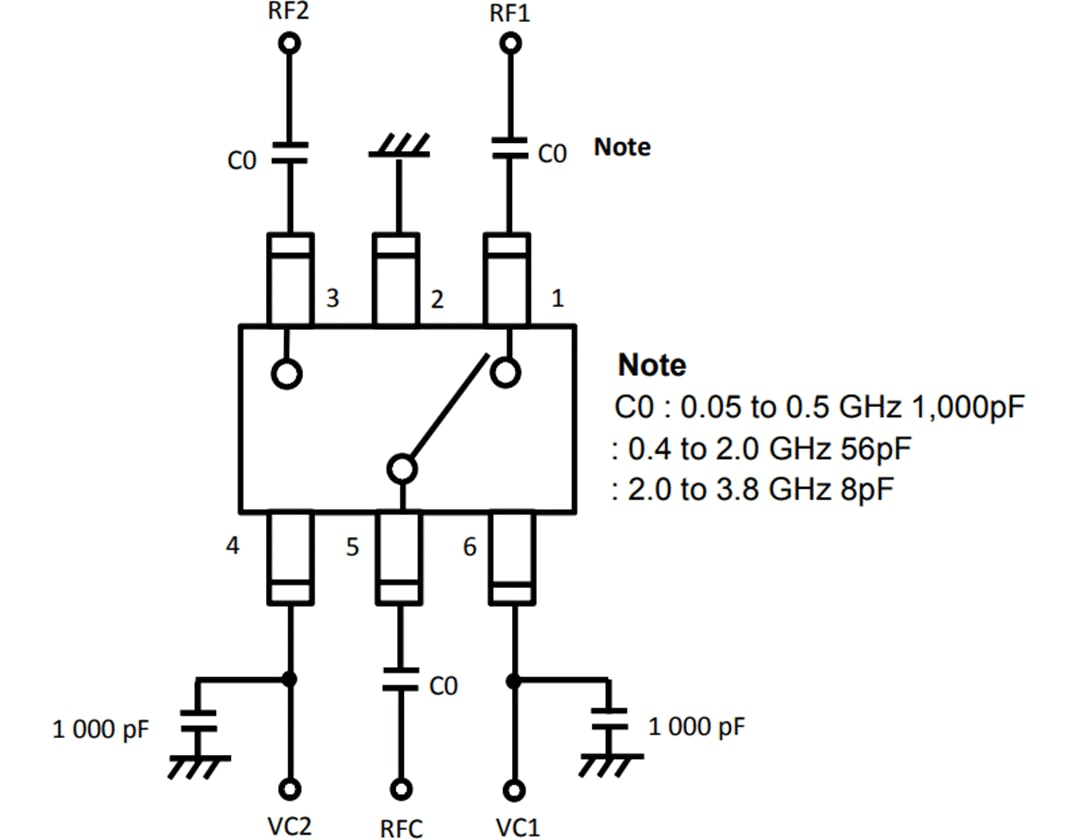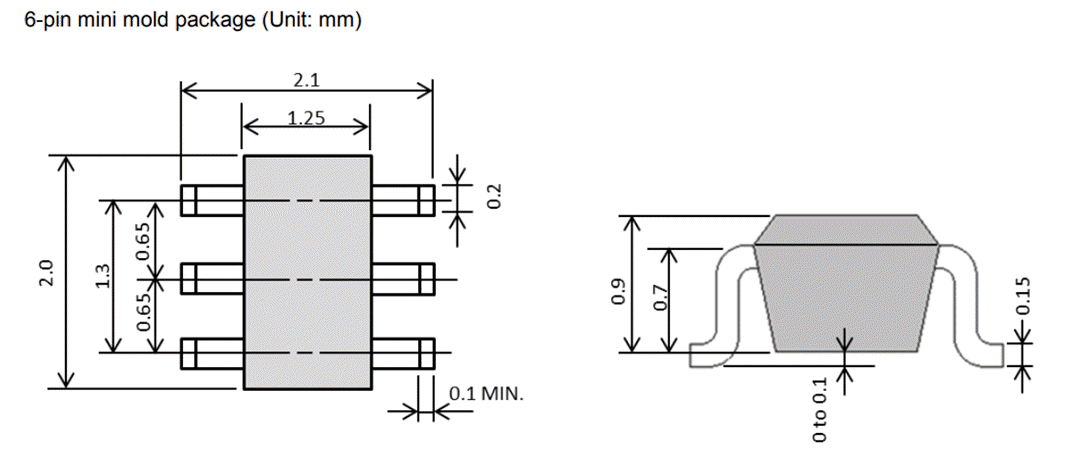# CEL CG2409M2 GaAs MMIC SPDT RF Switch

CEL CG2409M2 is a GaAs (Gallium arsenide) MMIC (Monolithic Microwave Integrated Circuit) High Power SPDT RF Switch IC designed for WiMAX and Wireless LAN applications. CG2409M2 operates within a -45°C to 85°C  temperature range with the voltage supply varying from 1.8V to 5V. The CG2409M2-C4 has low insertion loss and high isolation and is housed in a compact 6-pin mini mold package, ideal for space-constrained applications.

## Features

• Control voltage:
• VC (H) = 1.8 to 5V (3V TYPICAL)
• VC (L) = -0.2 to 0.2V (0V TYPICAL)
• Power Handling
• Pin (0.1dB) = +36.5dBm TYPICAL @ f = 0.4 to 3.8GHz, VC (H) = 3V, VC (L) = 0V
• Low insertion loss:
• Lins1 = 0.35dB TYPICAL @ f = 1GHz
• Lins2 = 0.42dB TYPICAL @ f = 2.5GHz
• Lins3 = 0.45dB TYPICAL @ f = 3GHz
• High isolation:
• ISL1 = 34dB TYPICAL @ f = 1GHz
• ISL2 = 30dB TYPICAL @ f = 2.5GHz
• ISL3 = 26dB TYPICAL @ f = 3GHz
• Pb-Free and RoHS compliant
• Package:
• 6-pin Mini Mold
• 2.0mm x 1.25mm x 0.9mm

## Applications

• Wireless LAN (IEEE802.11 b/g/n)
• WiMAX  (IEEE 802.16)

## Application Circuit## Package DimensionsPublished: 2017-05-11 | Updated: 2022-03-11